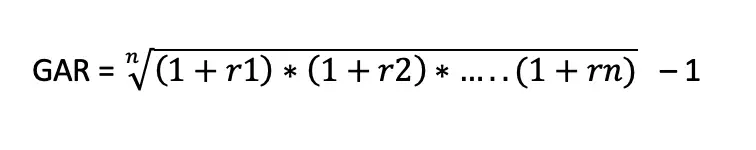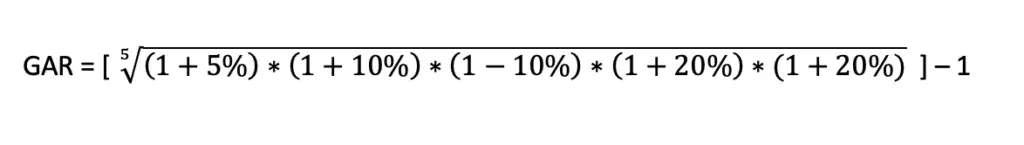# Geometric Average Return

Geometric Average Return or the compound annual return is the calculation of the average return of investment from beginning up to now. It simply means the average return over investment life from the first year. It is mostly used for investments that are compound over multiple periods.

We simply group similar investments into a portfolio and evaluate their performance together.  It focuses on the return generate over the period of time and it is compounded from one period to the next. It is the average rate of return that get from the total return and divided by the number of periods.

This rate is more accurate as it takes into account all return over the investment lifetime. While the other ratio uses only one year of return.

## Geometric Average Return FormulaGAR: Geometric Average Return

r: rat of return

n: number of periods

## Geometric Average Example

Company A has made an investment in a project which generate a return as follows:

Year Return
1 5%
2 10%
3 (10%)
4 20%
5 20%GAR = 8%

The geometric average return shows us the average return of this investment from year 1 to year 5 which is %

The arithmetic return simply combines all the return and divide by the number of years. The average return should be 5% (25% / 5).